# Precalculus : Tangents To a Curve

## Example Questions

2 Next →

### Example Question #6 : Find The Equation Of A Line Tangent To A Curve At A Given Point

Write the equation for the tangent line to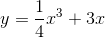at.

Possible Answers: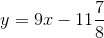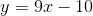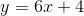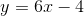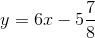Correct answer:Explanation:

First, find the slope of the tangent line by taking the first derivative: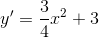To finish determining the slope, plug in the x-value, 2: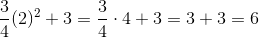the slope is 6

Now find the y-coordinate where x is 2 by plugging in 2 to the original equation: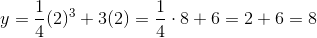To write the equation, start in point-slope form and then use algebra to get it into slope-intercept like the answer choices.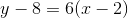distribute the 6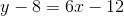add 8 to both sides### Example Question #7 : Find The Equation Of A Line Tangent To A Curve At A Given Point

Write the equation for the tangent line for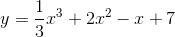at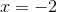.

Possible Answers: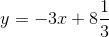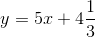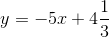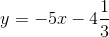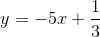Correct answer:Explanation:

First, take the first derivative in order to find the slope: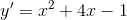To continue finding the slope, plug in the x-value, -2: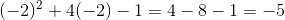Then find the y-coordinate by plugging -2 into the original equation: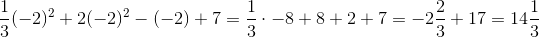The y-coordinate is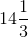Now write the equation in point-slope form then algebraically manipulate it to match one of the slope-intercept forms of the answer choices.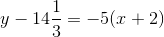distribute the -5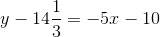addto both sides### Example Question #8 : Find The Equation Of A Line Tangent To A Curve At A Given Point

Write the equation for the tangent line to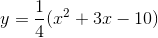at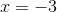.

Possible Answers: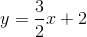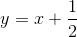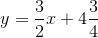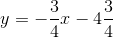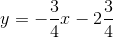Correct answer:Explanation:

First distribute the. That will make it easier to take the derivative: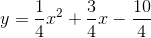Now take the derivative of the equation: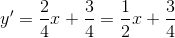To find the slope, plug in the x-value -3: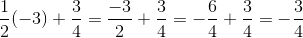To find the y-coordinate of the point, plug in the x-value into the original equation: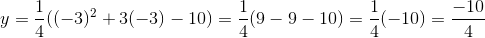Now write the equation in point-slope, then use algebra to get it into slope-intercept like the answer choices: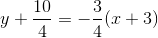distribute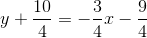subtract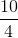from both sides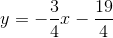write as a mixed number2 Next →

### All Precalculus Resources